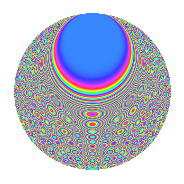# Properties

 Label 2100.2.cbLevel 2100 Weight 2 Character orbit cb Rep. character $$\chi_{2100}(41,\cdot)$$ Character field $$\Q(\zeta_{10})$$ Dimension 320 Sturm bound 960

# Related objects

## Defining parameters

 Level: $$N$$ $$=$$ $$2100 = 2^{2} \cdot 3 \cdot 5^{2} \cdot 7$$ Weight: $$k$$ $$=$$ $$2$$ Character orbit: $$[\chi]$$ $$=$$ 2100.cb (of order $$10$$ and degree $$4$$) Character conductor: $$\operatorname{cond}(\chi)$$ $$=$$ $$525$$ Character field: $$\Q(\zeta_{10})$$ Sturm bound: $$960$$

## Dimensions

The following table gives the dimensions of various subspaces of $$M_{2}(2100, [\chi])$$.

Total New Old
Modular forms 1968 320 1648
Cusp forms 1872 320 1552
Eisenstein series 96 0 96

## Trace form

 $$320q - 4q^{7} + O(q^{10})$$ $$320q - 4q^{7} - 22q^{15} + 6q^{21} + 8q^{25} - 8q^{37} - 32q^{39} + 32q^{43} - 20q^{49} - 24q^{51} + 148q^{57} + 17q^{63} + 32q^{67} + 56q^{79} - 44q^{81} + 92q^{85} + 2q^{91} + 92q^{93} + 16q^{99} + O(q^{100})$$

## Decomposition of $$S_{2}^{\mathrm{new}}(2100, [\chi])$$ into newform subspaces

The newforms in this space have not yet been added to the LMFDB.

## Decomposition of $$S_{2}^{\mathrm{old}}(2100, [\chi])$$ into lower level spaces

$$S_{2}^{\mathrm{old}}(2100, [\chi]) \cong$$ $$S_{2}^{\mathrm{new}}(525, [\chi])$$$$^{\oplus 3}$$$$\oplus$$$$S_{2}^{\mathrm{new}}(1050, [\chi])$$$$^{\oplus 2}$$

## Hecke characteristic polynomials

There are no characteristic polynomials of Hecke operators in the database